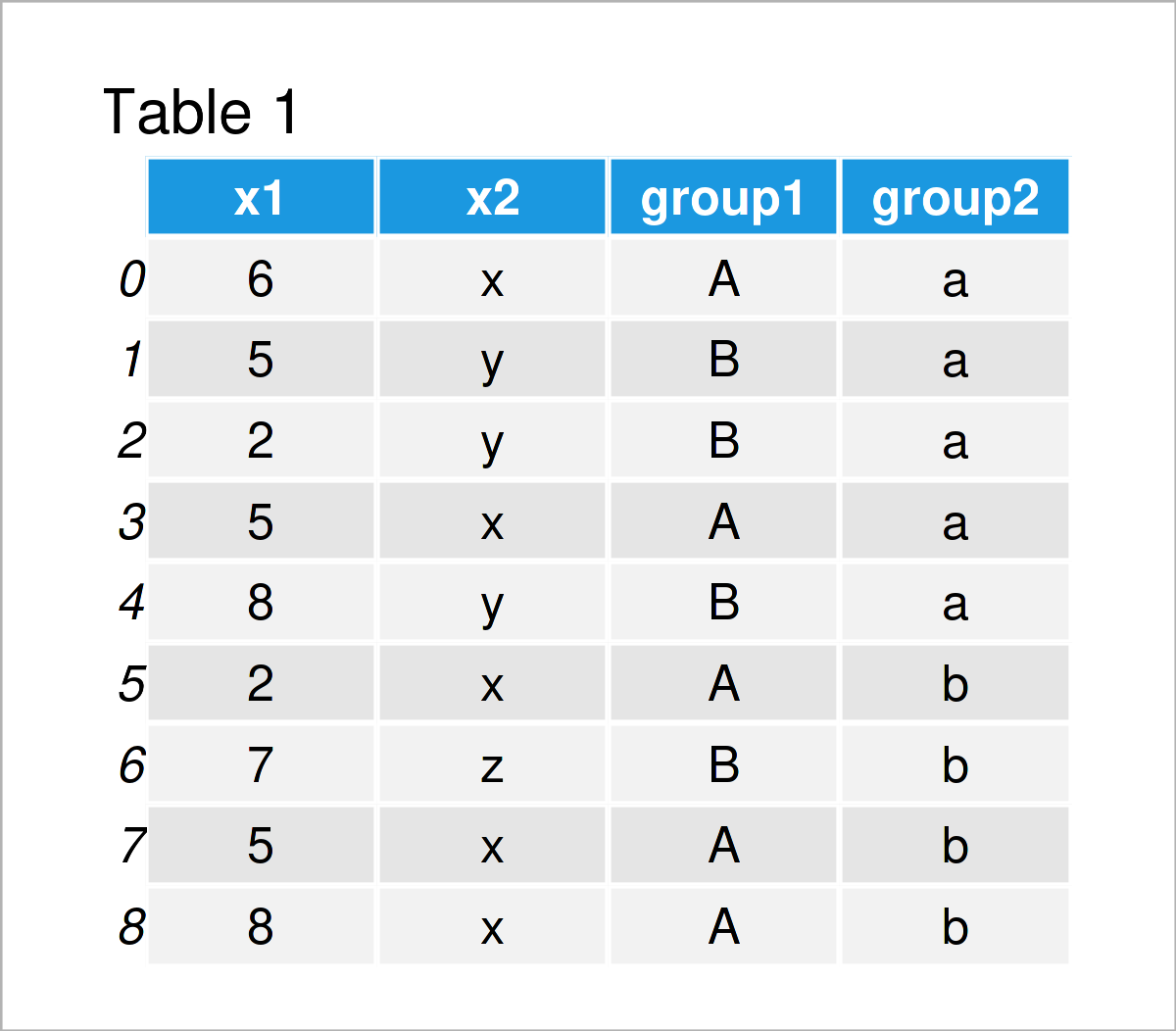# Calculate Mode by Group in Python (2 Examples)

In this tutorial, I’ll explain how to find the mode by group in the Python programming language.

You’re here for the answer, so let’s get straight to the examples:

## Example Data & Add-On Libraries

First, we need to load the pandas library:

`import pandas as pd                                                 # Import pandas library in Python`

Let’s also construct some example data:

```data = pd.DataFrame({'x1':[6, 5, 2, 5, 8, 2, 7, 5, 8],              # Create pandas DataFrame
'x2':['x', 'y', 'y', 'x', 'y', 'x', 'z', 'x', 'x'],
'group1':['A', 'B', 'B', 'A', 'B', 'A', 'B', 'A', 'A'],
'group2':['a', 'a', 'a', 'a', 'a', 'b', 'b', 'b', 'b']})
print(data)                                                         # Print pandas DataFrame```Table 1 shows the structure of our example pandas DataFrame: It consists of nine rows and four columns.

## Example 1: Mode by Group in pandas DataFrame

In this example, I’ll demonstrate how to GroupBy a pandas DataFrame and select the most common element (i.e. the mode) in Python.

To accomplish this, we have to use the groupby, agg, and value_counts functions as shown in the following Python code:

```print(data.groupby('group1').agg(lambda x:x.value_counts().index)) # Get mode by group
#         x1 x2 group2
# group1
# A        5  x      b
# B        8  y      a```

The previous output shows the modes for each group and every column in our data set.

## Example 2: Mode by Group & Subgroup in pandas DataFrame

The Python programming code below explains how to use multiple columns of a pandas DataFrame to create even smaller subgroups for the calculation of the mode.

For this task, we have to specify a list of all group indicators within the groupby function:

```print(data.groupby(['group1', 'group2']).agg(lambda x:x.value_counts().index)) # Get mode by multiple groups
#                x1 x2
# group1 group2
# A      a        5  x
#        b        8  x
# B      a        8  y
#        b        7  z```

The previous console output illustrates the mode values for each subgroup.

## Video & Further Resources

In case you need further explanations on the contents of this tutorial, I can recommend watching the following video on my YouTube channel. I show the Python programming syntax of this article in the video:

Furthermore, you might want to read the other articles on my homepage:

You have learned in this post how to compute the mode by group in the Python programming language. In case you have further questions and/or comments, let me know in the comments section below. Furthermore, please subscribe to my email newsletter in order to get updates on new tutorials.

Subscribe to the Statistics Globe Newsletter